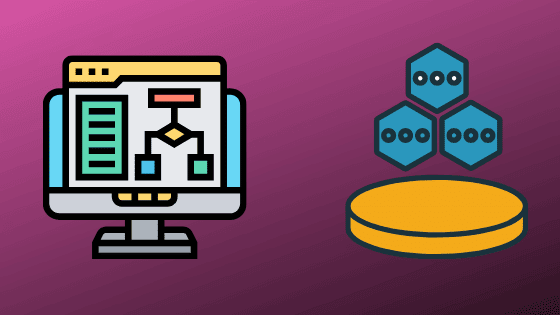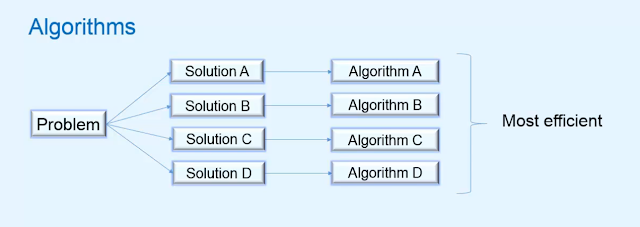What is data structure and algorithms: we are a programmer and we always work on a program of million lines of code. and to develop a program we need two important things.
• represent data in an efficient way.
• develop a step by step process.

## What is Data structures and algorithms?### How to represent data efficiently

to represent the data in an efficient way we only need a thing that is data structures.

### What are the data structures?

the data structure is nothing but a data representation method. there are many data structures available right now like an array, linked list, trees, heap, stack, queue, etc.

each data structure has its own way of implementation and own characteristics. and we need to choose an efficient data structure to represent our data in the program. because it makes a huge difference to choose the right data structure for our program.

#### how we can choose the right data structure for our program?

to choose the right data structure for our program we need to learn about different data structures and their characteristics. because it' only comes with practice and without the practice of data structure we don't predict which data structure perfectly to represent our data.

especially the characteristics of a specific data structure show that where are they used and when we choose a specific data structure to work on.

let's understand with an example:

if we can say we are working on a program in that we are working with linear data. so which data structure we need to choose. so we definitely choose arrays or single linked lists because the array can represent linear data efficiently or it's also true for a single linked list.

but if we select the wrong data type then it badly affects our code. and maybe it affects the complexity of our code. and a programmer always works to reduce the complexity of code. and that's why we are called a programmer.

so I think now you understand why we need to learn about data structure to develop the program.

others article for you

### How to develop a step by step procedure.

to develop a step by step procedure of any problem we need an algorithm. and an algorithm is nothing but a step by step procedure that contains well-set steps for performing a task.

to develop an algorithm first we need to choose a data structure. because after choosing a data structure we work on that data structure to perform a specific task. like sorting data, searching in data, etc.

similarly is true for the data structure. we use an algorithm for various operations on the data structure.

let's take a brief introduction on the algorithm

### What is the algorithm?

as we know that algorithm is nothing but a step by step procedure to solve any particular problem.

let's take an example to understand what is the algorithm.

let's say you need a cup of tea and by chance, you know how to make a tea. so how would you make tea?  so the process that you follow to make a cup of tea is nothing but an algorithm to make tea.

similarly in programming to develop a program that can perform a specific task we need an algorithm.

let's say you want to make a program in that you enter 10 input values and in the output you want to print all 10 values in ascending order. so how would you do that? to perform that specific task we need a step by step process that can take  10 different values as input and print these 10 values in ascending order.but the problem is that to solve a particular problem we have thousands of solutions. and for every solution, we have a specific algorithm. and among these algorithms which one we will select. to select and algorithm, we need to consider a thing that is complexity. the algorithm that has minimum complexity to solve a particular problem will be selected.

#### what is complexity?

The complexity of an algorithm is nothing but the running time that an algorithm needs to run and space that an algorithm needs to store in memory.

#### complexity has two types.

• Time complexity
• Space complexity

#### Time complexity

the time complexity of an algorithm is nothing but the time that an algorithm needs to run to solve a particular task.

let's say we apply an algorithm to print 10 different numbers in ascending order. and that algorithm takes 0.33 seconds to run and print the output. so 0.33 seconds is the time complexity of that algorithm.

#### Space complexity

The space complexity of an algorithm is nothing but the memory space an algorithm needs to store the defined variables and values in memory.

let's take the above same example to print 10 different numbers in ascending order. and that algorithm needs to take 30 bytes of memory space to store defined variables and values in memory. so 30 bytes is the space complexity of that algorithm.

#### How to select an algorithm.

we select an algorithm that has minimum time complexity and space complexity to perform a specific task.